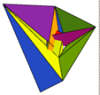Union College Department of Mathematics WeBWorK Homework Server

WeBWorK courses for Fall term 2023:

 Math 110 - 1 & 2 Calculus I Prof. Gasparovic Math 110 - 3 Calculus I Prof. Tonnesen-Friedman Math 110 - 4 Calculus I Prof. Wang Math 110 - 5 Calculus I Prof. Hatley Math 113 - 1 & 2 Accelerated Single-Variable Calculus Prof. Lesh Math 113 - 3 Accelerated Single-Variable Calculus Prof. Gajek-Leonard Math 113 - 4 Accelerated Single-Variable Calculus Prof. Jauregui Math 115 - 1 Calculus III Prof. Qian Math 115H - 1 Calculus III - Enriched Prof. Hatley Math 117 - 1 Calculus IV Prof. Friedman

Courses from past terms
An Introduction to WeBWork for Students

Union College Department of Mathematics